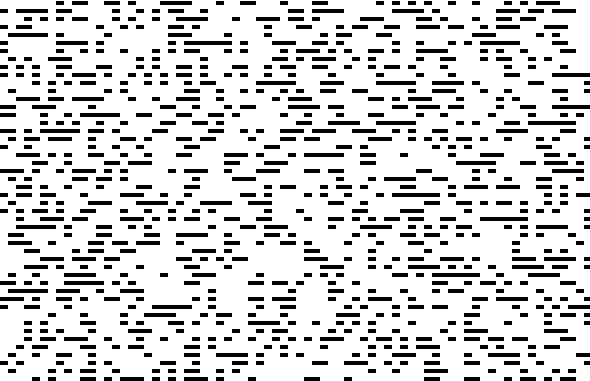# The Game of Life with Non-Local Neighbors

Oguz Yetkin and Clint Sprott
Department of Physics, University of Wisconsin, Madison, WI 53706, USA
April 23, 1998

Introduction and Background

Since its invention by Conway in the 1970s, the Game of Life has fascinated most everyone who has come across it. The game is a very simple simulation of life. The "world" is a rectangular N x M grid of live and dead cells. The fate of each cell in the next generation is determined by its eight nearest neighbors by the following rules:

• If a dead cell has exactly 3 neighbors, it is "born".
• If a living cell has < 3 neighbors, it dies of loneliness.
• If a living cell has 3 or 4 neighbors, it stays alive.
• If a living cell has > 4 neighbors, it dies of overcrowding.
As the generations are advanced, these simple rules lead to relatively complex behavior. The world typically settles down into a stable pattern (or periodic behavior) after at most several thousand iterations, depending on the size of the grid. Here's the code for a straightforward implementation in C++. If you'd like to play the game, here's a much better Windows implementation by someone else.

The Experiment

The game of life was modified so that neighbors of a cell could be assigned randomly. The rules governing birth and death remain the same with respect to the eight neighbors, which can now be anywhere in the grid. Such a model might mimic an organism that interacts mostly with other organisms not in its physical vicinity, such as an Internet community. You can download and examine the code for this new implementation:

In order to verify that the new implementation was correct, a test-case was run forcing the neighbors to be local. This implementation does indeed behave like the game of life.
Observations
1. More cells are alive after a large number of generations in the non-local neighbor version than in the version where the neighbors are forced to be local (37% vs. 2.5 %).
2. Small arrays usually end up having no living cells after 100 or 200 generations with the new implementation.
3. Making it legal for cells to have themselves as neighbors does not appear to have an important effect.
Here's a sample of the output for a case with 3600 grid points after 5404 generations:(More screen shots and code are available online.)

Discussion

The game of life with non-local neighbors eventually settles down to a probability density of around 37% (i.e., 37% of the cells are alive after a large number of iterations). This figure can be statistically justified as follows:

Let:

A = number of alive cells

D = number of dead cells

P = probability that a site is alive (= A / (A + D))

Pn = probability of a site having exactly n living neighbors

Nb = number born in each generation (= DP3)

Nd = number dying in each generation (= A(P0 + P1 + P4 + P5 + P6 + P7 + P8))

Since the probabilities P0 through P8 must sum to 1, the death rate can be simplified to
Nd = A(1 - P2 - P3)
The probability of having exactly n living neighbors can be calculated as follows:
P0 = (1 - P)8
P1 = 8(1 - P)7P
P2 = 28(1 - P)6P2
P3 = 56(1 - P)5P3
P4 = 70(1 - P)4P4
P5 = 56(1 - P)3P5
P6 = 28(1 - P)2P6
P7 = 8(1 - P)P7
P8 = P8
(The coefficients are 8 "choose" n, or "8 nCr n" for scientific calculator users.)

In equilibrium, the birth rate Nb must equal the death rate Nd, so that

DP3 = A(1 - P2 - P3)
We can now can solve for P
P = A / (A + D) = A / (A + A(1 - P2 - P3) / P3)) = P3 / (1 - P2)
Substituting the expressions for P3 and P2 leads to the following 8th order polynomial equation:
P8 - 8P7 + 25P6 + 150P5 + 35P4 - 16P3 + 3P2 - 1/28 = 0
This equation can be easily solved by the Newton-Raphson method, which gives the following four real roots for P:
-0.0872576258413151
0.192468887447466
0.370173837503678
3.00012395926967
There are presumably also two pairs of complex conjugate roots which are unphysical.  The first (negative) root above is unphysical as is the third root that gives a probability that exceeds 1.  Of the remaining roots, the one at 0.1924... is unstable, and the one at 0.3701... is stable and is in excellent agreement with numerical results for the Game of Life with (random) non-local neighbors.

Suggestions for Further Study

This study could be extended in a number of ways.  As with the regular Game of Life, you could alter the rules for the number of live sites required for birth and death.  You could look at neighborhoods smaller or larger than 8.  You could examine a hybrid scheme in which near neighbors are replaced by random far neighbors with a probability p.  This modification would more realistically represent many real-world processes such as social interactions, the spread of diseases, the electrical power grid, and the operation of the brain.